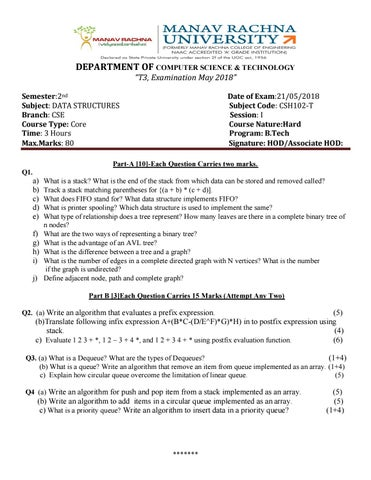DEPARTMENT OF COMPUTER SCIENCE & TECHNOLOGY “T3, Examination May 2018” Semester:2nd Subject: DATA STRUCTURES Branch: CSE Course Type: Core Time: 3 Hours Max.Marks: 80

Date of Exam:21/05/2018 Subject Code: CSH102-T Session: I Course Nature:Hard Program: B.Tech Signature: HOD/Associate HOD:

Part-A -Each Question Carries two marks. Q1.

a) b) c) d) e) f) g) h) i) j)

What is a stack? What is the end of the stack from which data can be stored and removed called? Track a stack matching parentheses for {(a + b) * (c + d)]. What does FIFO stand for? What data structure implements FIFO? What is printer spooling? Which data structure is used to implement the same? What type of relationship does a tree represent? How many leaves are there in a complete binary tree of n nodes? What are the two ways of representing a binary tree? What is the advantage of an AVL tree? What is the difference between a tree and a graph? What is the number of edges in a complete directed graph with N vertices? What is the number if the graph is undirected? Define adjacent node, path and complete graph? Part B Each Question Carries 15 Marks (Attempt Any Two)

Q2. (a) Write an algorithm that evaluates a prefix expression.

(5) (b)Translate following infix expression A+(B*C-(D/E^F)*G)*H) in to postfix expression using stack. (4) c) Evaluate 1 2 3 + *, 1 2 – 3 + 4 *, and 1 2 + 3 4 + * using postfix evaluation function. (6)

Q3. (a) What is a Dequeue? What are the types of Dequeues? (1+4) (b) What is a queue? Write an algorithm that remove an item from queue implemented as an array. (1+4) c) Explain how circular queue overcome the limitation of linear queue. (5) Q4 (a) Write an algorithm for push and pop item from a stack implemented as an array.

(b) Write an algorithm to add items in a circular queue implemented as an array. c) What is a priority queue? Write an algorithm to insert data in a priority queue?

*******

(5) (5) (1+4)

Part C Each Question Carries 15 Marks (Attempt Any Two) Q5.(a) Construct the binary tree with the following. (5) Inorder:- D H B E A F C I G Postorder:- H D E B F I G C A (b) What is a strictly binary tree? What is a complete binary tree? Is a complete binary tree a strictly binary tree too ? (2+2+1) c) What is an AVL tree? What are the types of rotations? (1+4) Q6.(a) Define the properties of B-tree? How does a B-tree differ from a binary tree? (2+1) (b) What is threaded binary tree? Explain the procedure to remove all the null pointers of binary tree.(5) c) What is binary search tree? Create a binary search tree for the numbers: 40,60,50,33,55,11. Find the inorder, preorder and postorder traversal of created binary search tree. (1+3+3) Q7. (a) Explain the difference between a directed and an undirected graph with example ? (5) (b) Represent a directed graph with nodes A, B, C, and D and edges (A, B), (C, D), (C, A), and (A,D) as a set of linked lists. (5) c) What are the searching techniques for graph? Describe depth first search algorithm (5)

*******